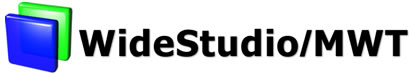WideStudio
Programming Guide
WideStudio Index

How to select the key events on the input field

You can select or reject/convert the key events on the input field with the event procedure by the WSEV_KEY_HOOK trigger.

```(* A WSEV_KEY_HOOK sample procedure. *)
(* Set the input field with the WSEV_KEY_HOOK trigger. *)
...

let hookop obj =
let key = get_int (_WSGIappKeyboard '() -> getKey () ) in
if (((key >= get_int _WSK_0) && ( key < get_int _WSK_9)) ||
((key >= get_int _WSK_KP_0) && ( key < get_int _WSK_KP_9)) ||
(key = get_int _WSK_plus)  || (key = get_int _WSK_minus) ||
(key = get_int _WSK_BackSpace) || (key = get_int _WSK_Delete) || (key =
get_int _WSK_Insert) ||
(key = get_int _WSK_space) || (key = get_int _WSK_Up) || (key = get_int
_WSK_Down) ||
(key = get_int _WSK_Left) || (key = get_int _WSK_Right) || (key = get_int
_WSK_Return) ) then begin
(* dispatches.. *)
end else begin
(* Reject the other. *)
ignore(_WSGIappKeyboard '() -> setKey (0));
end
()

let _ = Callback.register "hookop" hookop
```

This sample of the input field shows how to choose the key event. _WSGIappKeyboard '()->setKey(0) reject the key event which you do not need.

Document Release 3.80 for WideStudio ver 3.80, Jan 2005